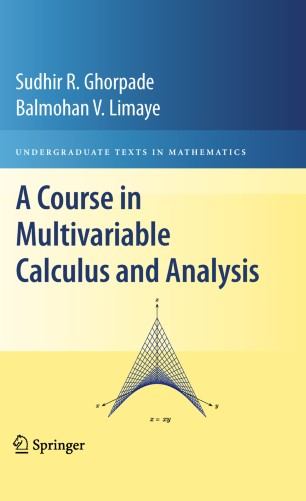# A Course in Multivariable Calculus and Analysis

• Balmohan V. LimayeTextbook

Part of the Undergraduate Texts in Mathematics book series (UTM)

1. Front Matter
Pages I-XII
2. Sudhir R. Ghorpade, Balmohan V. Limaye
Pages 1-42
3. Sudhir R. Ghorpade, Balmohan V. Limaye
Pages 43-82
4. Sudhir R. Ghorpade, Balmohan V. Limaye
Pages 83-156
5. Sudhir R. Ghorpade, Balmohan V. Limaye
Pages 157-184
6. Sudhir R. Ghorpade, Balmohan V. Limaye
Pages 185-290
7. Sudhir R. Ghorpade, Balmohan V. Limaye
Pages 291-368
8. Sudhir R. Ghorpade, Balmohan V. Limaye
Pages 369-462
9. Sudhir R. Ghorpade, Balmohan V. Limaye
Pages C1-C7
10. Back Matter
Pages 465-477

### Introduction

This self-contained textbook gives a thorough exposition of multivariable calculus. It can be viewed as a sequel to the one-variable calculus text, A Course in Calculus and Real Analysis, published in the same series. The emphasis is on correlating general concepts and results of multivariable calculus with their counterparts in one-variable calculus. For example, when the general definition of the volume of a solid is given using triple integrals, the authors explain why the shell and washer methods of one-variable calculus for computing the volume of a solid of revolution must give the same answer. Further, the book includes genuine analogues of basic results in one-variable calculus, such as the mean value theorem and the fundamental theorem of calculus.

This book is distinguished from others on the subject: it examines topics not typically covered, such as monotonicity, bimonotonicity, and convexity, together with their relation to partial differentiation, cubature rules for approximate evaluation of double integrals, and conditional as well as unconditional convergence of double series and improper double integrals. Moreover, the emphasis is on a geometric approach to such basic notions as local extremum and saddle point.

Each chapter contains detailed proofs of relevant results, along with numerous examples and a wide collection of exercises of varying degrees of difficulty, making the book useful to undergraduate and graduate students alike. There is also an informative section of "Notes and Comments’’ indicating some novel features of the treatment of topics in that chapter as well as references to relevant literature. The only prerequisite for this text is a course in one-variable calculus.

### Keywords

Convexity Derivative Fundamental theorem of calculus Implicit function calculus continuity cubature rules differential equation double integrals double series extrema improper double integrals mean value theorem monotonicity multivariable calculus